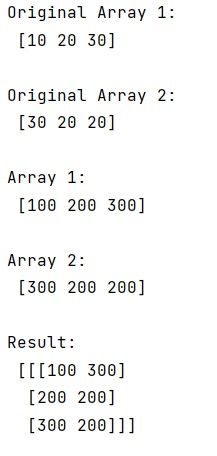# NumPy: Multiply array with scalar

Learn, how to multiply a NumPy array with a scalar value in Python?
Submitted by Pranit Sharma, on February 19, 2023

NumPy is an abbreviated form of Numerical Python. It is used for different types of scientific operations in python. Numpy is a vast library in python which is used for almost every kind of scientific or mathematical operation. It is itself an array which is a collection of various methods and functions for processing the arrays.

## Multiplying NumPy array with scalar

Suppose that we are given a numpy array and a specific scalar value which we need to multiply with our given array. After multiplying the scalar value with the numpy array, we need to stack these arrays along the third axis.

For this purpose, we will directly multiply the numpy array with the specific scalar value and then we will use the numpy.dstack() method.

The numpy.dstack() method is used to stack arrays in sequence depth-wise (along the third axis). It takes a parameter called tup which is the sequence of arrays and It returns an array formed by stacking the given arrays.

Let us understand with the help of an example,

## Python code to multiply a NumPy array with a scalar value

```# Import numpy
import numpy as np

# Creating two numpy arrays
arr1 = np.array([10, 20, 30])
arr2 = np.array([30, 20, 20])

# Display original arrays
print("Original Array 1:\n",arr1,"\n")
print("Original Array 2:\n",arr2,"\n")

# Defining a scalar value
sc = 10

# Multiplying arrays with sc
arr1 = arr1 * sc
arr2 = arr2 * sc

# Display Modified arrays
print("Array 1:\n",arr1,"\n")
print("Array 2:\n",arr2,"\n")

# Using dstack method
res = np.dstack((arr1,arr2))

# Display result
print("Result:\n",res,"\n")
```

Output: